# Treewidth and Integer Programming Mixed Integer Programming workshop

• Slides: 33Treewidth and Integer Programming Mixed Integer Programming workshop June 5, 2006, Miami Arie. Koster Zuse Konrad-Zuse-Zentrum Institute Berlin (ZIB) für Informationstechnik Berlin (ZIB) Zuse Institute (ZIB) Joint work with Adrian Zymolka (ZIB) [email protected] de http: //www. zib. de/koster/ http: //www. zib. de/koster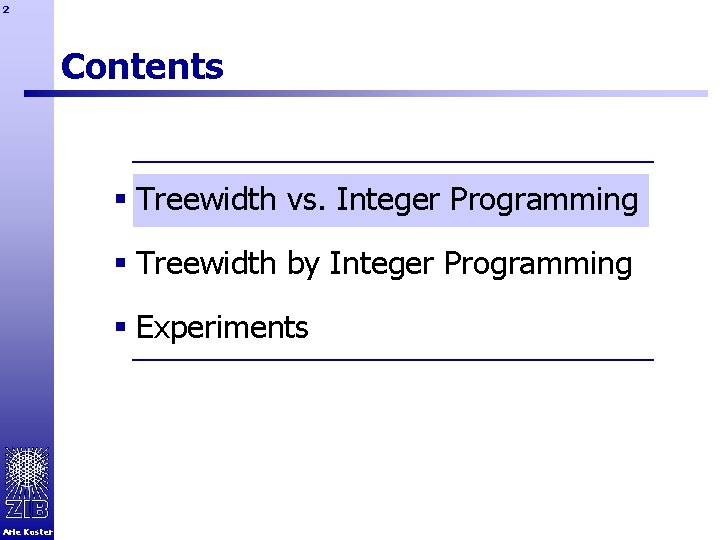2 Contents § Treewidth vs. Integer Programming § Treewidth by Integer Programming § Experiments Arie Koster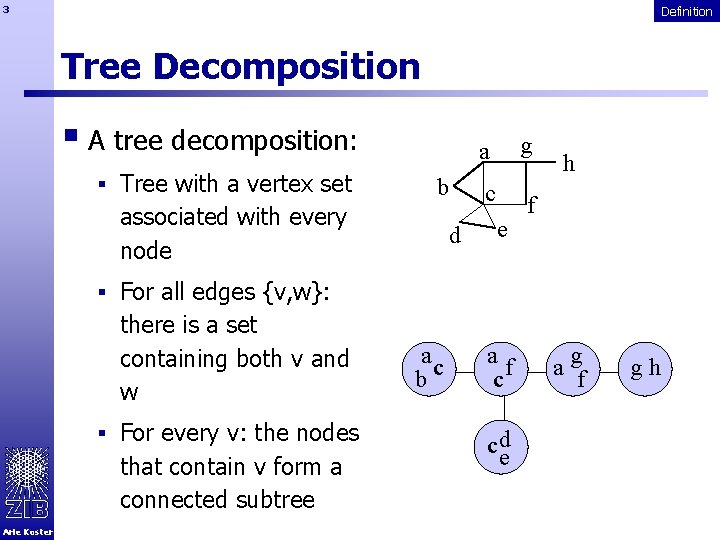3 Definition Tree Decomposition § A tree decomposition: § Tree with a vertex set b associated with every node d a g c f e h § For all edges {v, w}: there is a set containing both v and w § For every v: the nodes that contain v form a connected subtree Arie Koster ac b af c c ed ag f gh4 Definition Tree Decomposition § A tree decomposition: § Tree with a vertex set b associated with every node d a g c f e h § For all edges {v, w}: there is a set containing both v and w § For every v: the nodes that contain v form a connected subtree Arie Koster ac b af c c ed ag f gh5 Definition Tree Decomposition § A tree decomposition: § Tree with a vertex set b associated with every node d a g c f e h § For all edges {v, w}: there is a set containing both v and w § For every v: the nodes that contain v form a connected subtree Arie Koster ac b af c c de a gf gh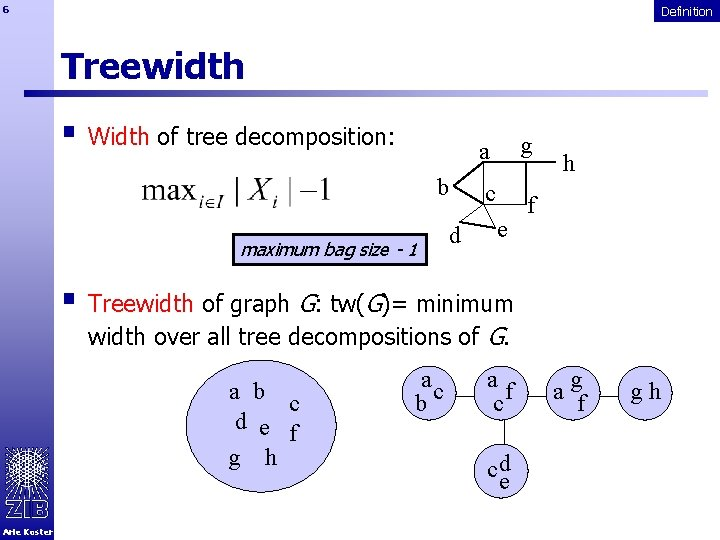6 Definition Treewidth § Width of tree decomposition: b maximum bag size - 1 d a g c f e h § Treewidth of graph G: tw(G)= minimum width over all tree decompositions of G. a b c d e f g h Arie Koster ac b af c c ed ag f gh7 Definition First observations g a b c d e h f ac b af c a gf c ed Each clique has to be part of at least one node Clique number - 1 is a lower bound for treewidth Trees have treewidth 1 Arie Koster gh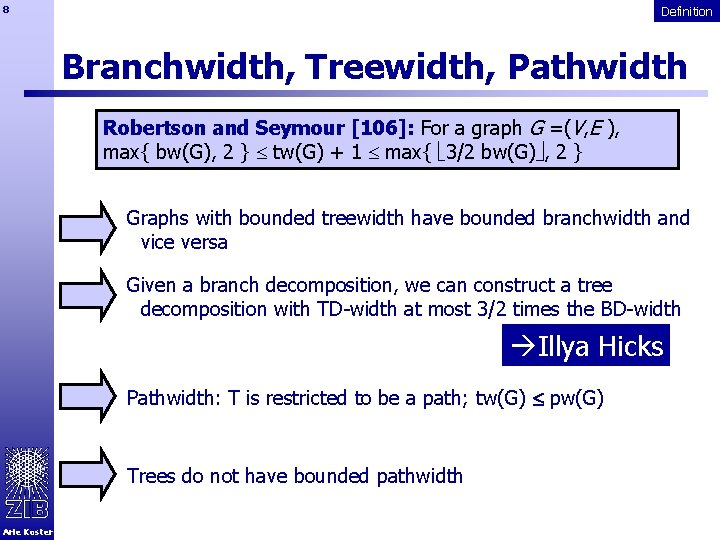8 Definition Branchwidth, Treewidth, Pathwidth Robertson and Seymour : For a graph G =(V, E ), max{ bw(G), 2 } tw(G) + 1 max{ 3/2 bw(G) , 2 } Graphs with bounded treewidth have bounded branchwidth and vice versa Given a branch decomposition, we can construct a tree decomposition with TD-width at most 3/2 times the BD-width àIllya Hicks Pathwidth: T is restricted to be a path; tw(G) pw(G) Trees do not have bounded pathwidth Arie Koster9 Algorithms using tree decompositions TD-based Algorithms § Step 1: Find a tree decomposition of width bounded by some small k. § Heuristics. § O(f(k)n) in theory. § Fast O(n) algorithms for k=2, k=3. § By construction, e. g. , for trees, series-parallel-graphs. § Step 2. Use dynamic programming, bottom-up on the tree. § Let Yi= Xi over all descendants of i I § Compute optimal solution in G[Yi] for each set S Xi, based on the solutions for the children Arie Koster10 TD-based Algorithms Maximum weighted independent set on graphs with treewidth k § For node i in tree decomposition, S Xi write § R(i, S) = maximum weight of independent set Xi = S, – ¥ if such S does not exist S of G[Yi] with S § Compute for each node i, a table with all values R(i, …). § Each such table can be computed in O(2 k) time when treewidth at most k. § Gives O(n) algorithm when treewidth is (small) constant. Many problems can be solved in polynomial time given a graph of bounded treewidth § Probabilistic networks § Frequency assignment Arie Koster11 TD-based Algorithms Minimum Interference FAP § Graph G=(V, E) § Vertices correspond to bi-directional connections § Edges indicate interference between two connections § For every vertex v, set of frequency pairs D(v) is specified § Interference quantified by edge penalties p(v, f , w, g) § Preferences for frequencies quantified by penalties q(v, f) § Objective: Select for each vertex exactly one frequency, such that the total penalty is minimized. Arie Koster12 TD-based Algorithms Does it work in practice ? § Only with (pre)processing techniques § Graph reduction § Vertices with degree 1 can be removed § Vertices with degree 2 can be removed § Domain reduction § Upper bounding § Dominance of domain elements Arie Koster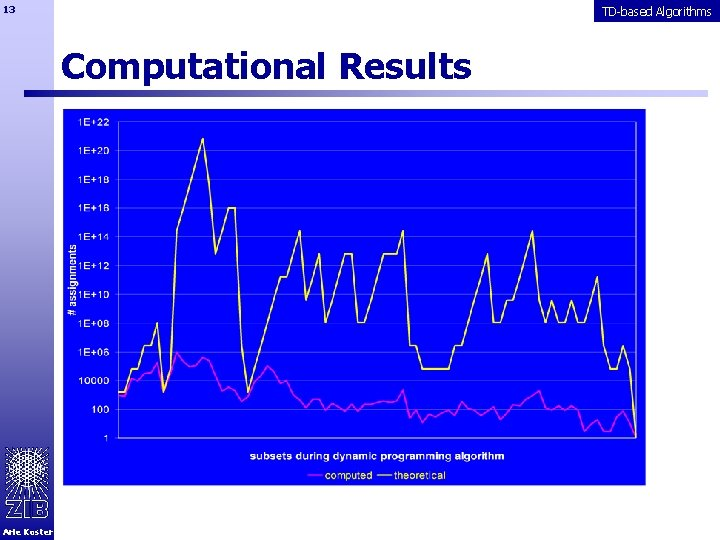13 TD-based Algorithms Computational Results Arie Koster14 How do we get a tree decomposition all small width? Computing Treewidth TREEWIDTH: Given k 0 and G a graph, is the treewidth of G k ? Computing TREEWIDTH is NP-hard Arnborg et al.  Linear time algorithm for TREEWIDTH if k not part of the input Bodlaender  § Exponential in k § Not practical, even for k as small as 4 Several exponential time algorithms § O( 2 n poly(n) ) § O( 1. 9601 n poly(n) ) § poly(n) denotes a polynomial in n Arie Koster Arnborg et a Fomin et al.  References refer to Tutorials 2005 chapter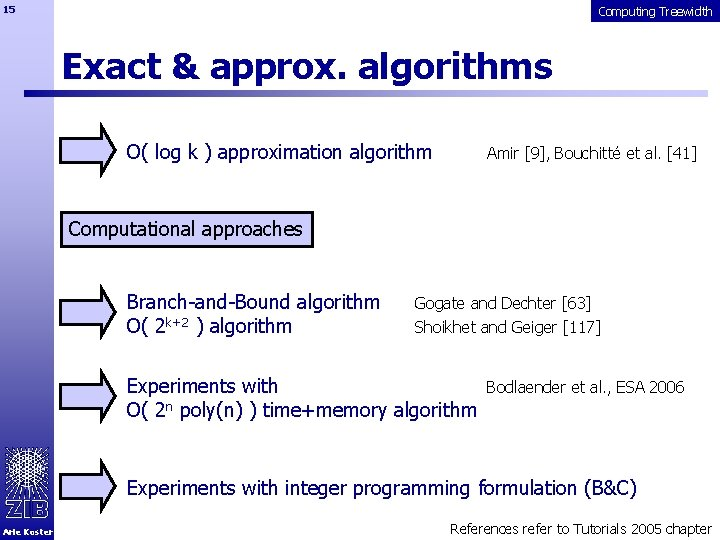15 Computing Treewidth Exact & approx. algorithms O( log k ) approximation algorithm Amir , Bouchitté et al.  Computational approaches Branch-and-Bound algorithm O( 2 k+2 ) algorithm Gogate and Dechter  Shoikhet and Geiger  Experiments with O( 2 n poly(n) ) time+memory algorithm Bodlaender et al. , ESA 2006 Experiments with integer programming formulation (B&C) Arie Koster References refer to Tutorials 2005 chapter16 Computing Treewidth Other approaches àHeuristic algorithms based on chordal graphs àMinimum separating set heuristic  àMetaheuristics § Tabu Search  § Simulated Annealing  § Genetic algorithm  àPreprocessing § Reduction rules  § Safe Separators  Arie Koster References refer to Tutorials 2005 chapter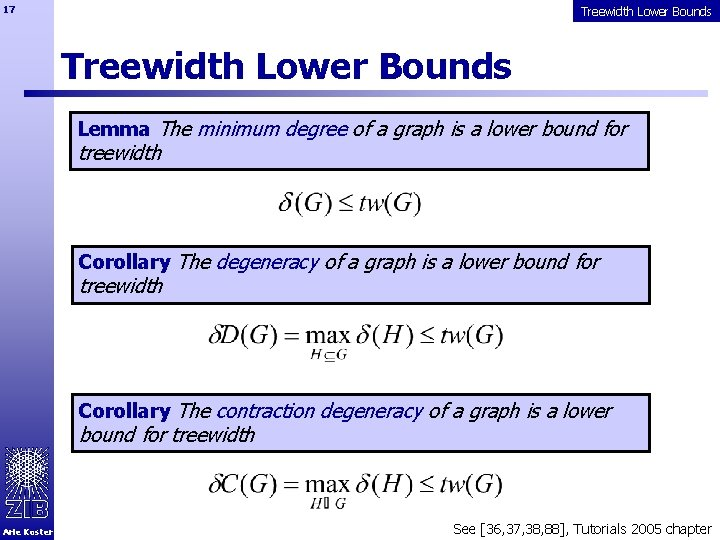17 Treewidth Lower Bounds Lemma The minimum degree of a graph is a lower bound for treewidth Corollary The degeneracy of a graph is a lower bound for treewidth Corollary The contraction degeneracy of a graph is a lower bound for treewidth Arie Koster See [36, 37, 38, 88], Tutorials 2005 chapter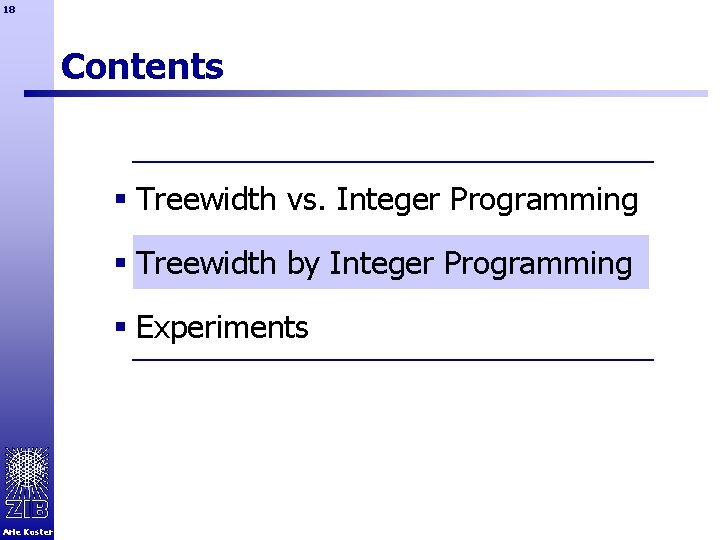18 Contents § Treewidth vs. Integer Programming § Treewidth by Integer Programming § Experiments Arie Koster19 Treewidth by IP ? Chordal graphs Chordal graph: Every cycle of size at least 4 contains a chord Gavril (1974): A graph G =(V, E ) is chordal if and only if there exists a tree T =(I, F ) such that one can associate with each vertex v V a subtree Tv=(Iv, Fv ) of T, such that vw E if and only if Iv Iw . There exists a chordalization H =(V, E F ) of G with maximum clique size k+1 if and only if the treewidth of G is k. Let H(G ) be the set of all chordalizations of G. Select best H and compute maximum clique size! Arie Koster20 Related questions Fill-in: Minimum #edges to be added to obtain a chordal graph. There exists a chordalization H =(V, E F ) of G with |F | = k if and only if the fill-in of G is k. Weighted treewidth (weights c(v)): Minimum over all tree decompositions of the maximum product v Xic(v) over all bags i I. There exists a chordalization H =(V, E F ) of G with maximum clique product k if and only if the weighted treewidth of G is k. Arie Koster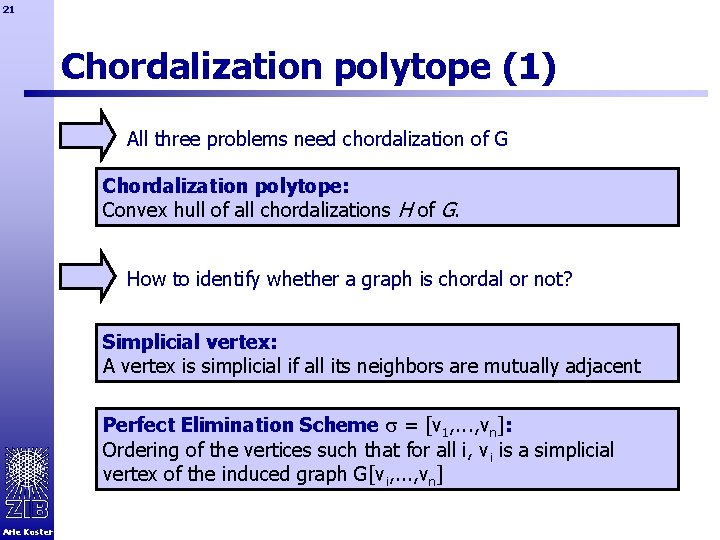21 Chordalization polytope (1) All three problems need chordalization of G Chordalization polytope: Convex hull of all chordalizations H of G. How to identify whether a graph is chordal or not? Simplicial vertex: A vertex is simplicial if all its neighbors are mutually adjacent Perfect Elimination Scheme = [v 1, . . . , vn]: Ordering of the vertices such that for all i, vi is a simplicial vertex of the induced graph G[vi, . . . , vn] Arie Koster22 Chordalization polytope (2) Existence of edges Simplicity of vertices Ordering of vertices Arie Koster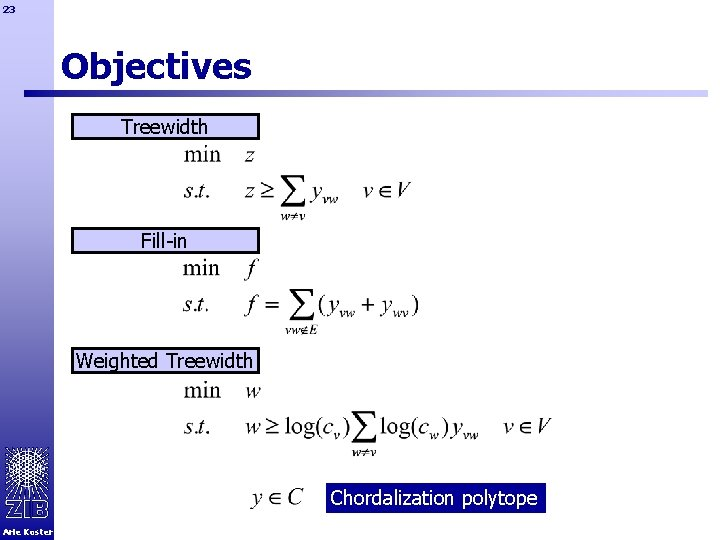23 Objectives Treewidth Fill-in Weighted Treewidth Chordalization polytope Arie Koster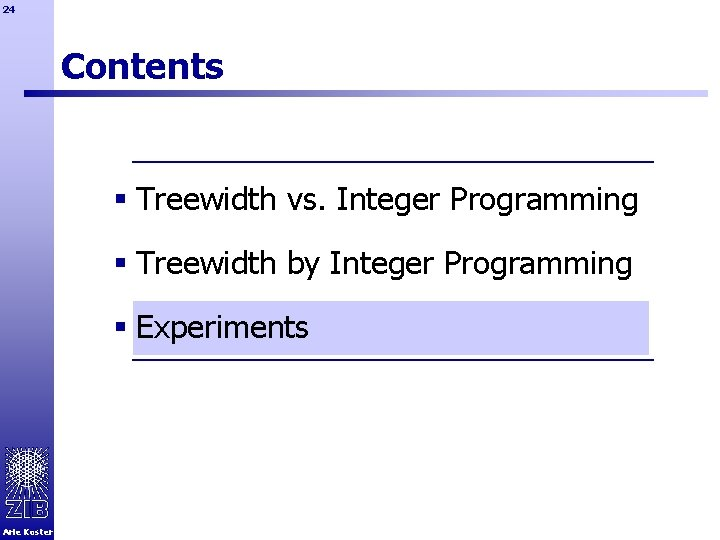24 Contents § Treewidth vs. Integer Programming § Treewidth by Integer Programming § Experiments Arie Koster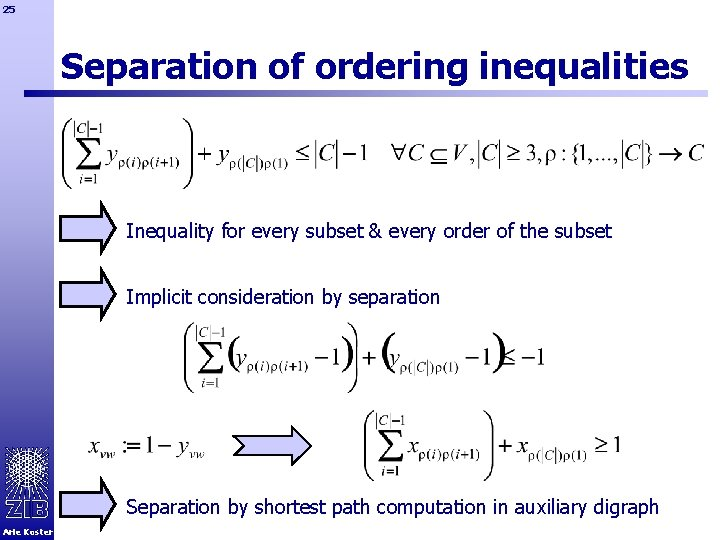25 Separation of ordering inequalities Inequality for every subset & every order of the subset Implicit consideration by separation Separation by shortest path computation in auxiliary digraph Arie Koster26 Simplicity of vertices Inequality for every triple of vertices Always satisfied if vw E Other implicitly handled by separation (lazy cuts) Arie Koster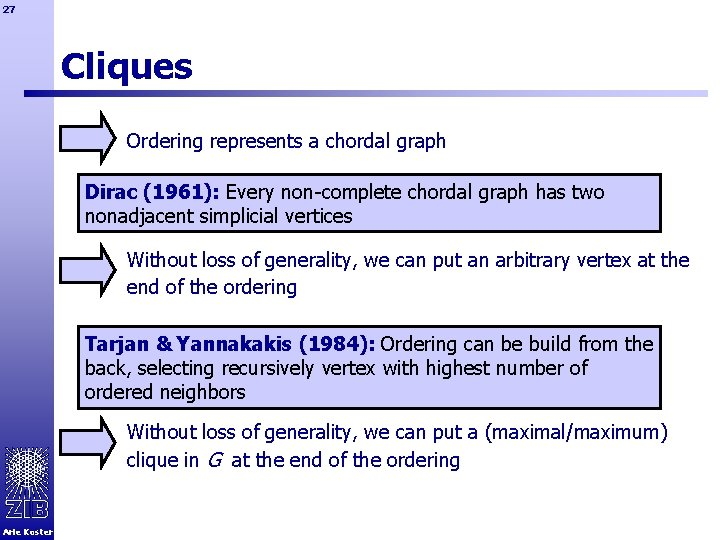27 Cliques Ordering represents a chordal graph Dirac (1961): Every non-complete chordal graph has two nonadjacent simplicial vertices Without loss of generality, we can put an arbitrary vertex at the end of the ordering Tarjan & Yannakakis (1984): Ordering can be build from the back, selecting recursively vertex with highest number of ordered neighbors Without loss of generality, we can put a (maximal/maximum) clique in G at the end of the ordering Arie Koster28 Instances Randomly generated partial-k-trees (Shoiket&Geiger, 1998) § Generate k-tree § Randomly remove p% of the edges àtreewidth at most k àn=100, k=10, p=30/40/50 Instances from frequency assignment, probabilistic networks, … Computational framework SCIP (http: //scip. zib. de/) with CPLEX 10. 0 as LP solver Arie Koster29 Petersen graph Objective Strategy Treewidth none Treewidth maximum clique Fill-in none Fill-in maximum clique CPU time (s) B&C nodes Gap (%) 449. 18 278018 0 0. 43 57 0 >3600 >886765 41. 18 1. 27 379 0 Maximum clique breaks symmetries(? ); simplifies computation Fill-in more difficult than treewidth? ? ? Arie Koster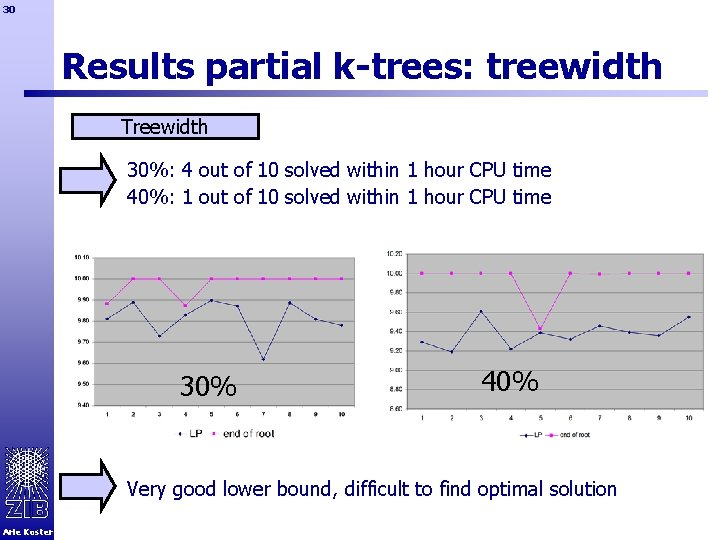30 Results partial k-trees: treewidth Treewidth 30%: 4 out of 10 solved within 1 hour CPU time 40%: 1 out of 10 solved within 1 hour CPU time 30% 40% Very good lower bound, difficult to find optimal solution Arie Koster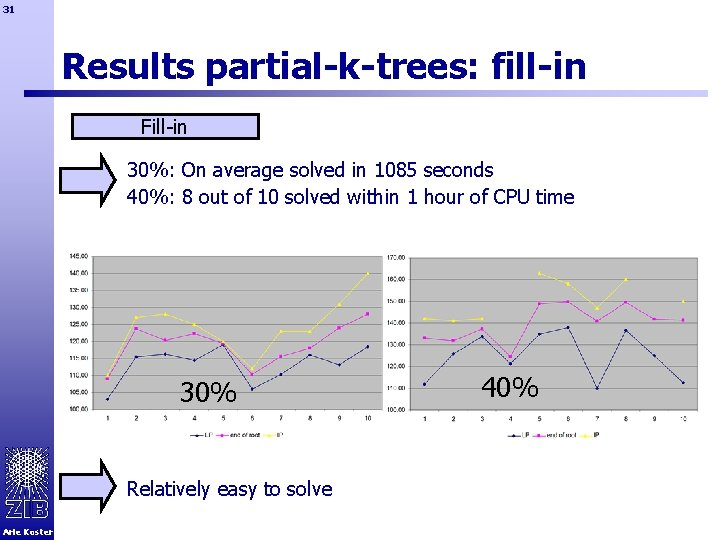31 Results partial-k-trees: fill-in Fill-in 30%: On average solved in 1085 seconds 40%: 8 out of 10 solved within 1 hour of CPU time 30% Relatively easy to solve Arie Koster 40%32 Results realistic instances minors of link-pp selected; (G)=9, tw(G)=13 treewidth Arie Koster CPU(s) #nodes fill-in CPU(s) Combined instance |V| |E| fi(G) #nodes CPU(s) #nodes link-pp-minor-020 20 125 29 23. 42 9680 0. 86 2 4. 88 1307 link-pp-minor-021 21 130 35 29. 91 7238 1. 29 9 13. 15 2767 link-pp-minor-022 22 137 38 37. 82 5858 1. 33 1 7. 88 349 link-pp-minor-023 23 144 40 128. 21 16131 2. 25 2 15. 22 986 link-pp-minor-024 24 151 43 399. 61 27125 1. 93 2 103. 50 8568 link-pp-minor-025 25 156 48 1875. 24 94369 3. 61 3 133. 67 6861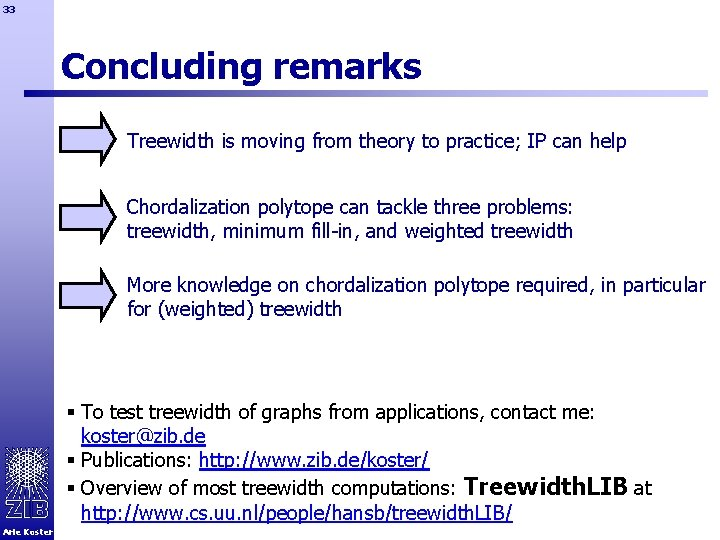33 Concluding remarks Treewidth is moving from theory to practice; IP can help Chordalization polytope can tackle three problems: treewidth, minimum fill-in, and weighted treewidth More knowledge on chordalization polytope required, in particular for (weighted) treewidth § To test treewidth of graphs from applications, contact me: [email protected] de § Publications: http: //www. zib. de/koster/ § Overview of most treewidth computations: Treewidth. LIB at http: //www. cs. uu. nl/people/hansb/treewidth. LIB/ Arie Koster